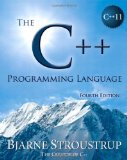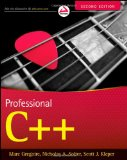# C++14 lambda tutorial

## Posted on August 28, 2014 by Paul

The last iteration of C++, C++14 was approved this month. C++14 brings a few much anticipated changes to the C++11 standard, like allowing auto to be used as the return type of a function, or generic lambdas - the subject of this article.

Lambdas, in C++, were introduced by the C++11 standard. They were meant to let the coder write short, anonymous functions, that could be used instead of a function object, avoiding the need to create a separate class and a function definition. Here is a typical example of C++11 lambda usage, that returns the square of a number:

If you need to reuse the same piece of code in more than one place, you can save the function in a variable:

Can you see the problem with the above code ? It can be used only for integers. What if, we want to use the same lambda for a double or for a complex number ? Say that we want to be able to write something like this:

We could obviously use a function template:

However, this is not the solution we are looking for in the context of this article, the above is a named, global function,

The C++14 standard introduced the concept of generalized lambda, basically we are allowed to use auto for the parameter types of a lambda expression. We could write a shorter and, in a way, more elegant solution now:

Here is a complete program that exemplifies the above:

The above code can be compiled with any modern C++ compiler, like GCC 4.9.x or Clang. Here is the result of compiling and running the code with GCC and Clang on OS X:

However, the generic lambda shines in combination with STL. Suppose that you want to sort a vector in decreasing order. Using a generic lambda, we can write:

Here is a complete example of sorting a vector, of ten integers, in decreasing order, using generic lambda and the STL:

This is the result of running the above code on my machine:

If you are interested to learn more about the new C++11 syntax I would recommend reading The C++ Programming Language by Bjarne Stroustrup.or, Professional C++ by M. Gregoire, N. A. Solter, S. J. Kleper: